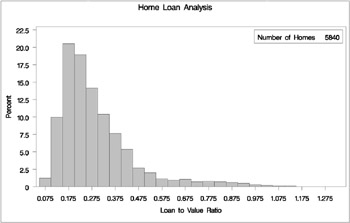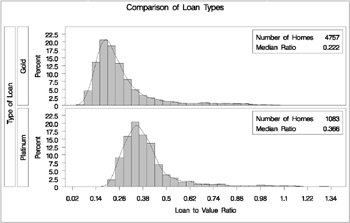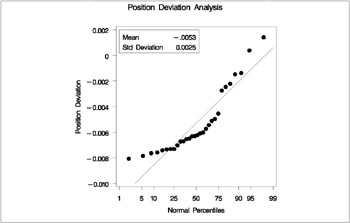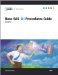# Getting Started

The following examples demonstrate how you can use the UNIVARIATE procedure to analyze the distributions of variables through the use of descriptive statistical measures and graphical displays, such as histograms.

### Capabilities of PROC UNIVARIATE

The UNIVARIATE procedure provides a variety of descriptive measures, high-resolution graphical displays, and statistical methods , which you can use to summarize, visualize, analyze, and model the statistical distributions of numeric variables. These tools are appropriate for a broad range of tasks and applications:

• Exploring the distributions of the variables in a data set is an important preliminary step in data analysis, data warehousing, and data mining. With the UNIVARIATE procedure you can use tables and graphical displays, such as histograms and nonparametric density estimates, to find key features of distributions, identify outliers and extreme observations, determine the need for data transformations, and compare distributions.

• Modeling the distributions of data and validating distributional assumptions are basic steps in statistical analysis. You can use the UNIVARIATE procedure to fit parametric distributions (beta, exponential, gamma, lognormal, normal, and Weibull) and to compute probabilities and percentiles from these models. You can assess goodness of fit with hypothesis tests and with graphical displays such as probability plots and quantile-quantile plots. You can also use the UNIVARIATE procedure to validate distributional assumptions for other types of statistical analysis. When standard assumptions are not met, you can use the UNIVARIATE procedure to perform nonparametric tests and compute robust estimates of location and scale.

• Summarizing the distribution of the data is often helpful for creating effective statistical reports and presentations. You can use the UNIVARIATE procedure to create tables of summary measures, such as means and percentiles, together with graphical displays, such as histograms and comparative histograms, which facilitate the interpretation of the report.

The following examples illustrate a few of the tasks that you can carry out with the UNIVARIATE procedure.

### Summarizing a Data Distribution

Figure 3.1 shows a table of basic summary measures and a table of extreme observations for the loan-to-value ratios of 5,840 home mortgages. The ratios are saved as values of the variable LoanToValueRatio in a data set named HomeLoans . The following statements request a univariate analysis:

`  ods select BasicMeasures ExtremeObs;   proc univariate data=homeloans;   var LoanToValueRatio;   run;  ``  The UNIVARIATE Procedure   Variable: LoanToValueRatio (Loan to Value Ratio)   Basic Statistical Measures   Location                   Variability   Mean     0.292512    Std Deviation           0.16476   Median   0.248050    Variance                0.02715   Mode     0.250000    Range                   1.24780   Interquartile Range     0.16419   Extreme Observations   -------Lowest------       -----Highest-----   Value     Obs           Value      Obs   0.0651786       1         1.13976     5776   0.0690157       3         1.14209     5791   0.0699755      59         1.14286     5801   0.0702412      84         1.17090     5799   0.0704787       4         1.31298     5811  `Figure 3.1: Basic Measures and Extreme Observations

The ODS SELECT statement restricts the default output to the tables for basic statistical measures and extreme observations.

The tables in Figure 3.1 show, in particular, that the average ratio is 0.2925 and the minimum and maximum ratios are 0.06518 and 1.1398, respectively.

### Exploring a Data Distribution

Figure 3.2 shows a histogram of the loan-to-value ratios. The histogram reveals features of the ratio distribution, such as its skewness and the peak at 0.175, which are not evident from the tables in the previous example. The following statements create the histogram:

`  title 'Home Loan Analysis';   proc univariate data=homeloans noprint;   histogram LoanToValueRatio / cfill=ltgray;   inset n = 'Number of Homes' / position=ne;   run;  `Figure 3.2: Histogram for Loan-to-Value Ratio

The NOPRINT option suppresses the display of summary statistics. The INSET statement inserts the total number of analyzed home loans in the northeast corner of the plot.

The data set HomeLoans contains a variable named LoanType that classifies the loans into two types: Gold and Platinum. It is useful to compare the distributions of LoanToValueRatio for the two types. The following statements request quantiles for each distribution and a comparative histogram, which are shown in Figure 3.3 and Figure 3.4.

`  title 'Comparison of Loan Types';   ods select Quantiles MyHist;   proc univariate data=HomeLoans;   var LoanToValueRatio;   class LoanType;   histogram LoanToValueRatio / cfill=ltgray   kernel(color=black)   name='MyHist';   inset n='Number of Homes' median='Median Ratio' (5.3) / position=ne;   label LoanType = 'Type of Loan';   run;  ``  Comparison of Loan Types   The UNIVARIATE Procedure   Variable: LoanToValueRatio (Loan to Value Ratio)   LoanType = Gold   Quantiles (Definition 5)   Quantile        Estimate   100% Max       1.0617647   99%            0.8974576   95%            0.6385908   90%            0.4471369   75% Q3         0.2985099   50% Median     0.2217033   25% Q1         0.1734568   10%            0.1411130   5%             0.1213079   1%             0.0942167   0% Min         0.0651786   Comparison of Loan Types   The UNIVARIATE Procedure   Variable: LoanToValueRatio (Loan to Value Ratio)   LoanType = Platinum   Quantiles (Definition 5)   Quantile       Estimate   100% Max       1.312981   99%            1.050000   95%            0.691803   90%            0.549273   75% Q3         0.430160   50% Median     0.366168   25% Q1         0.314452   10%            0.273670   5%             0.253124   1%             0.231114   0% Min         0.215504  `Figure 3.3: Quantiles for Loan-to-Value RatioFigure 3.4: Comparative Histogram for Loan-to-Value Ratio

The ODS SELECT statement restricts the default output to the tables of quantiles. The CLASS statement specifies LoanType as a classification variable for the quantile computations and comparative histogram. The KERNEL option adds a smooth nonparametric estimate of the ratio density to each histogram. The INSET statement specifies summary statistics to be displayed directly in the graph.

The output in Figure 3.3 shows that the median ratio for Platinum loans (0.366) is greater than the median ratio for Gold loans (0.222). The comparative histogram in Figure 3.4 enables you to compare the two distributions more easily.

The comparative histogram shows that the ratio distributions are similar except for a shift of about 0.14.

A sample program, univar1.sas , for this example is available in the SAS Sample Library for Base SAS software.

### Modeling a Data Distribution

In addition to summarizing a data distribution as in the preceding example, you can use PROC UNIVARIATE to statistically model a distribution based on a random sample of data. The following statements create a data set named Aircraft containing the measurements of a position deviation for a sample of 30 aircraft components .

`  data Aircraft;   input Deviation @@;   label Deviation = 'Position Deviation';   datalines;   -.00653 0.00141 -.00702 -.00734 -.00649 -.00601   -.00631 -.00148 -.00731 -.00764 -.00275 -.00497   -.00741 -.00673 -.00573 -.00629 -.00671 -.00246   -.00222 -.00807 -.00621 -.00785 -.00544 -.00511   -.00138 -.00609 0.00038 -.00758 -.00731 -.00455   ;   run;  `

An initial question in the analysis is whether the measurement distribution is normal. The following statements request a table of moments, the tests for normality, and a normal probability plot, which are shown in Figure 3.5 and Figure 3.6:

`  title 'Position Deviation Analysis';   ods select Moments TestsForNormality MyPlot;   proc univariate data=Aircraft normaltest;   var Deviation;   probplot Deviation / normal (mu=est sigma=est)   square name='MyPlot';   label Deviation = 'Position Deviation';   inset mean std / format=6.4;   run;  ``  Position Deviation Analysis   The UNIVARIATE Procedure   Variable: Deviation (Position Deviation)   Moments   N                        30    Sum Weights               30   Mean             -0.0053067    Sum Observations     -0.1592   Std Deviation    0.00254362    Variance          6.47002E-6   Skewness          1.2562507    Kurtosis          0.69790426   Uncorrected SS   0.00103245    Corrected SS      0.00018763   Coeff Variation  -47.932613    Std Error Mean     0.0004644   Tests for Normality   Test                   --Statistic---   -----p Value------   Shapiro-Wilk           W     0.845364   Pr < W      0.0005   Kolmogorov-Smirnov     D     0.208921   Pr > D     <0.0100   Cramer-von Mises       W-Sq  0.329274   Pr > W-Sq  <0.0050   Anderson-Darling       A-Sq  1.784881   Pr > A-Sq  <0.0050  `Figure 3.5: Moments and Tests for NormalityFigure 3.6: Normal Probability Plot

All four goodness-of-fit tests in Figure 3.5 reject the hypothesis that the measurements are normally distributed.

Figure 3.6 shows a normal probability plot for the measurements. A linear pattern of points following the diagonal reference line would indicate that the measurements are normally distributed. Instead, the curved point pattern suggests that a skewed distribution, such as the lognormal, is more appropriate than the normal distribution.

A lognormal distribution for Deviation is fitted in Example 3.26.

A sample program, univar2.sas , for this example is available in the SAS Sample Library for Base SAS software.Base SAS 9.1 Procedures Guide, Volumes 1, 2, 3 and 4
ISBN: 1590472047
EAN: 2147483647
Year: 2004
Pages: 74